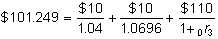﻿ 3.12 Constructing Your Own Yield Curveoffice (412) 967-9367
fax (412) 967-5958
toll-free 1 (800) 214-3480

3.12 Constructing Your Own Yield Curve

The relationship between the yield to maturity for zero-coupon securities (with no default risk) and the time to maturity is called the term structure of interest rates.

For the U.S. economy, the Treasury yield curve is obtained from the prices of Treasury strips. Strips are zero-coupon Treasury bonds, which are traded for a wide variety of maturities. From Treasury strip prices, you can directly obtain the yield curve.

For the coupon bonds in the very liquid Treasury bond and note markets, you will find interest rate quotations telling you the yields to maturity.

You know that except for zero-coupon bonds, the yield to maturity is not what you use to discount future cash flows. Instead, you should use the spot interest rates. You have also seen that any coupon bond can be engineered from a portfolio of zero-coupon bonds. This means that there is a well-defined relationship between the strip market and the bond market.

This leads to a question: If you know yields for coupon bonds, can you reconstruct the spot interest rates? We show you some different methods for this, based on the assumption that there is no arbitrage across the markets (i.e., the Treasury bonds and notes are priced in accordance with the spot interest rates).

There are at least two ways to reconstruct spot interest rates. One way is a rough approximation, and the second way requires all the details about each Treasury contract so that the timing and magnitude of all cash flows are known. In the first method, we assume that the yields to maturity on all bonds are approximately equal to the spot interest rates. The justification for this is that the face amount represents most of the cash flow. Of course, this result is more biased, the longer the life of the bond. The second method computes implied zero-coupon bond spot rates progressively, starting from the "short end" of the curve (i.e., rates for short maturities).

Fortunately for the second, more exact method, Treasury bills are traded at the short end. These securities are zero-coupon bonds. From this short end, the zero-coupon bond rates (i.e., spot interest rates) are calculated progressively for the sequence of longer-maturity notes and bonds. The cash flows from the note or bond are required to calculate progressive spot rates. This can be illustrated by a three year bond example.

Assume the three year term structure of interest rates is:

 Maturity 1+ Spot Rate 1 Year 1.0400 2 Year 1.0696 3 Year 1.0989

Using this yield curve, we can determine the price of several types of bonds:

1. Three year coupon bond (coupon rate 10%, compounded annually with face value equal to \$100);

2. Two year coupon bond (coupon rate 5%, compounded annually with face value equal to \$100);

3. One year zero-coupon bond (face value = \$100);

4. Two year zero-coupon bond (face value = \$100);

5. Three year zero-coupon bond (face value = \$100).

By using the true term structure of spot interest rates, each zero-coupon bond and the coupon bond can be valued at the beginning of Year 1 relative to the realized term structure.

The spot interest rates are 1.04, 1.0696, and 1.0989, respectively, and thus, the value of each security relative to the assumed term structure is determined as follows:

1. Coupon bond ((\$10/1.04)+(\$10/(1.0696^2))+(\$110/(1.0989^3)) = \$101.249

2. Coupon bond ((\$10/1.04)+(\$10/(1.0696^2))+(\$110/(1.0989^3)) = \$96.587

3. One year zero (\$100/1.04) = \$96.154

4. Two year zero (\$100/(1.0696^2)) = \$87.409

5. Three year zero (\$100/(1.0989^3)) = \$75.357

The yield to maturity for each security is:

1. 0.09502

2. 0.06884

3. 0.04000

4. 0.06960

5. 0.09890

Thus, the true yield curve can be obtained from the three zero-coupon bonds.

Method 1: Using Strips

Method 1 provides the yield curve immediately by plotting the strip yields to maturity against maturity. In the example, this is obtained directly from Securities 3, 4, and 5.

What if you can only observe Securities 1, 2, and 3? How would you compute the true yield curve?

Method 2: Approximate Method

Under Method 2, we assume that the yields to maturity for the coupon bonds equal the spot rates.

 Time Yield to Maturity Yield Curve Year 1 Zero Coupon 0.0400 0.0400 Year 2 Coupon bond 0.0688 0.0696 Year 3 Coupon bond 0.0950 0.0989

This approximate method understates the true yield curve, when the true curve is upward sloping. This is because of the averaging effect that earlier cash flows at lower spot rates have on the yield to maturity.

Method 3: Progressive Construction

The price of the two year coupon bond equals 96.587, and the cash flows are \$5 at the end of Year 1 and \$105 at the end of Year 2. We know the Year 1 spot rate, and thus can solve for the Year 2 spot rate as follows:Solving in this manner results in the appropriate two year spot rate, which equals 0.0696.

Similarly, from the price of the three year coupon bond we can solve for the three year spot rate:Solving now for the three year rate results in 0.0989.

As a result, the progressive method exactly recreates the true yield curve:

 Time Progressive Spot Rates Yield Curve Year 1 Zero Coupon 0.0400 0.0400 Year 2 Coupon bond 0.0696 0.0696 Year 3 Coupon bond 0.0989 0.0989

In practice, if the spot rates are computed from Treasury notes and bills, the convention of settling accrued interest, must be taken into account.